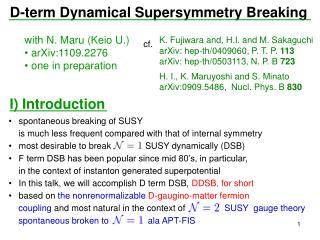Download PresentationD-term Dynamical Supersymmetry Breaking

# D-term Dynamical Supersymmetry Breaking - PowerPoint PPT PresentationDownload Presentation## D-term Dynamical Supersymmetry Breaking

- - - - - - - - - - - - - - - - - - - - - - - - - - - E N D - - - - - - - - - - - - - - - - - - - - - - - - - - -
##### Presentation Transcript

1. D-term Dynamical Supersymmetry Breaking • with N. Maru (Keio U.) • arXiv:1109.2276 • one in preparation K. Fujiwara and, H.I. andM. Sakaguchi arXiv: hep-th/0409060, P. T. P. 113 arXiv: hep-th/0503113, N. P. B 723 H. I., K. Maruyoshi and S. Minato arXiv:0909.5486, Nucl. Phys. B 830 cf. I) Introduction • spontaneous breaking of SUSY • is much less frequent compared with that of internal symmetry • most desirable to break SUSY dynamically (DSB) • F term DSB has been popular since mid 80’s, in particular, • in the context of instanton generated superpotential • In this talk, we will accomplish D term DSB, DDSB, for short • based on the nonrenormalizableD-gaugino-matter fermion • coupling and most natural in the context of SUSY gauge theory • spontaneous broken to alaAPT-FIS

2. II) Basic idea • Start from a general lagrangian • bilinears: where . no bosonic counterpart assume is the 2nd derivative of a trace fn. : a Kähler potential : a gauge kinetic superfield of the chiral superfield in the adjoint representation : a superpotential. : holomorphic and nonvanishing part of the mass the gauginos receive masses of mixed Majorana-Dirac type and are split.

3. Determination of stationary condition to where is the one-loop contribution and is a counterterm. condensation of the Dirac bilinear is responsible for In fact, the stationary condition is nothing but the well-known gap equation of the theory on-shell which contains four-fermi interactions.

4. The rest of my talk Contents I) Introduction II) Basic idea III) Illustrationby the Theory with vacuum at tree level IV) Mass spectrum at tree level and supercurrent V) Self-consistent HartreeFockapproximation VI)Vacuum shift and metastability(qualitative) VII) Our work in the context of MSSM VIII) More on the fermion masses in the H. F. (qualitative)

5. III) Theory with vacuum at tree level Action to work with • U(N) gauge group assumed for definiteness (product gauge group O.K.) • : prepotential, input function • superpotential W supplied by the electric and magnetic FI terms, • made possible by a particular fixing of rigid SU(2)R symmetry • should contrast with • Later, will work with

6. Off-shell component lagrangian The off-shell component lagrangian is where is the Kähler metric and its derivatives are defined as and . The gauge part is, in components, Finally, the superpotential can be written as

7. Eq of motion for auxiliary fields While, from the transformation laws,

8. susy of and tree vacua • construction of 2nd susy : Let be • the form of and are derived by imposing • ; vacuum condition • generic breaking pattern of gauge symmetry: so that follows from where 2nd susy broken

9. vacuum condition

10. V) Self-consistent Hartree-Fock approximation For simplicity, consider the case U(N) unbroken Recall we hunt for the possibility (up to one-loop): Mixed Maj.-Dirac mass to gaugino, no such coupling to bosons present DSB

11. the entire contribution to the 1PI vertex function • : mass matrix (holomorphic and nonvanishing part) The eigenvalues are We obtain where

12. : In order to trade A with in Vc.t. , impose, for instance, we obtain (some number),

13. is a stationary condition to Aside from a trivial solution , a nontrivial transcendental solution gap eq. In the approximate form in general exists • gap equation: susy is broken to .

14. VI) Vacuum shift and metastability(qualitative) • vacuum condition of vacuum computable • e.g.

15. obviously and • the tree vacuum is not lifted. • So the vacuum is metastable. • Estimate of the decay rate: provided can be made long lived

16. Symbolically • vector superfields, chiral superfields, their coupling • extend this to the type of actions with s-gluons and adjoint fermions • so as not to worry about mirror fermions e.t.s. • gauge group , the simplest case being • Due to the non-Lie algebraic nature of • the third prepotential derivatives, or , • we do not really need messenger superfields. VII) Our work in the context of MSSM

17. transmission of DDSB in to the rest of the theory by higher order • loop-corrections the sfermion masses Fox, Nelson, Weiner, JHEP(2002) the gaugino masses of the quadratic Casimir of representation some function of , which is essentially

18. Demanding • We obtain

19. VIII) More on the fermion masses in the H. F. (qualitative) • Back to the general theory with 3 input functions • H. F. can be made into a systematic expansion by an index loop argument. • Take to be . • In the unbroken phase, • The gap eq. is

20. Two sources beyond tree but leading in H. F. • i) Due to the vacuum shift, as well • ii) For U(1) sector, an index loop circulates + These contribute to the masses in the leading order in the H. F.

21. D-term Dynamical Supersymmetry Breaking • with N. Maru (Keio U.) • arXiv:1109.2276 • one in preparation K. Fujiwara and, H.I. andM. Sakaguchi arXiv: hep-th/0409060, P. T. P. 113 arXiv: hep-th/0503113, N. P. B 723 H. I., K. Maruyoshi and S. Minato arXiv:0909.5486, Nucl. Phys. B 830 cf. Obserbale (SU(N)) sector mass mass massive fermion scalar gluon gluino gluon -1/2 0 1/2 -1 -1/2 0 1/2 1